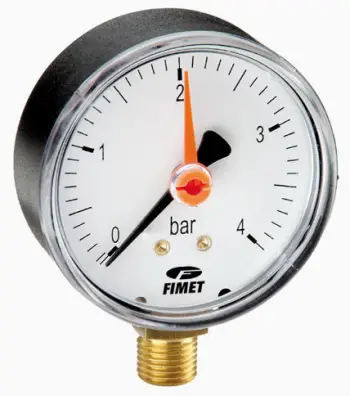# Definition of pressureIn everyday life we find many examples of pressure we often use: atmospheric pressure to talk about time changes, blood pressure to talk about health, pressure of the car's wheels in the engine field. We even talk about pressure on issues that have no link with physics: the pressure of a job interview.

Force and pressure are very different concepts. The pressure (symbol P) is the physical quantity that measures the instantaneous force in a unit of surface, applied in a direction perpendicular to it. Its unity in the SI is the Pascal. Pascal is equivalent to one Newton per square meter (N / m2). Other units can also be used to measure pressure such as mercury millimeter, bar and atmosphere.

The pressure can be expressed in terms of absolute pressure or relative pressure (also called normal pressure, gauge pressure or gauge pressure). The absolute pressure is the sum of the relative pressure and the atmospheric pressure. The standard atmospheric pressure is 101,325 Pa.

The pressure P indicates, for a given instant, the normal value of the force F at each point of a surface S.

## Units for measuring pressure

The unit of the international system for force is the Newton (N), for the surface is the square meter (m2), so, for the pressure will be the (N / m2). To this value, since it is used usually has been given a special name, Pascal (Pa), in honor to Blaise Pascal.

Pascal (Pa) is the unit of measurement of pressure in the International System. But it is a small unit, and other units of pressure measurement may sometimes be used:

Hectopascals (HPa). Used in meteorology. This unit, equals 100 Pa.
• Atmosphere (atm). It is a unit that indicates the pressure produced by the earth's atmosphere on average at the surface of the earth. To the pressure at this point we give the value of 1 atmosphere. The name of this unit will be atmosphere (atm) and equals 101,300 Pa.
• Kiloponds per square centimeter (kp / cm2). This unit of pressure is used in engineering. In this field, it is usually treated with very large weights which makes it not useful to speak of Newtons. The altrenativa is kilopond (kp). The kilopond is the weight of a mass of one kilogram, and therefore equals 9.8 N.

## Examples of pressure orders of magnitude

• 0.5 Pa Atmospheric pressure on Pluto
• 9.81 Pa Pressure caused by a depth of 1 mm of water
• 1 kPa Atmospheric pressure on Mars
• 10 kPa The pressure caused by a depth of 1 m of water, or the pressure difference between sea level and 1000 m height
• 101.325 kPa Atmospheric pressure at sea level
• 10 GPa Pressure needed for diamond formation, and some other minerals

## Devices for measurement of pressure

Among the different pressure measuring devices we find:

• The barometer, for measuring atmospheric pressure
• Manometer to measure pressure differences between two fluids.

valoración: 4 - votos 3

Last review: November 15, 2016

Back